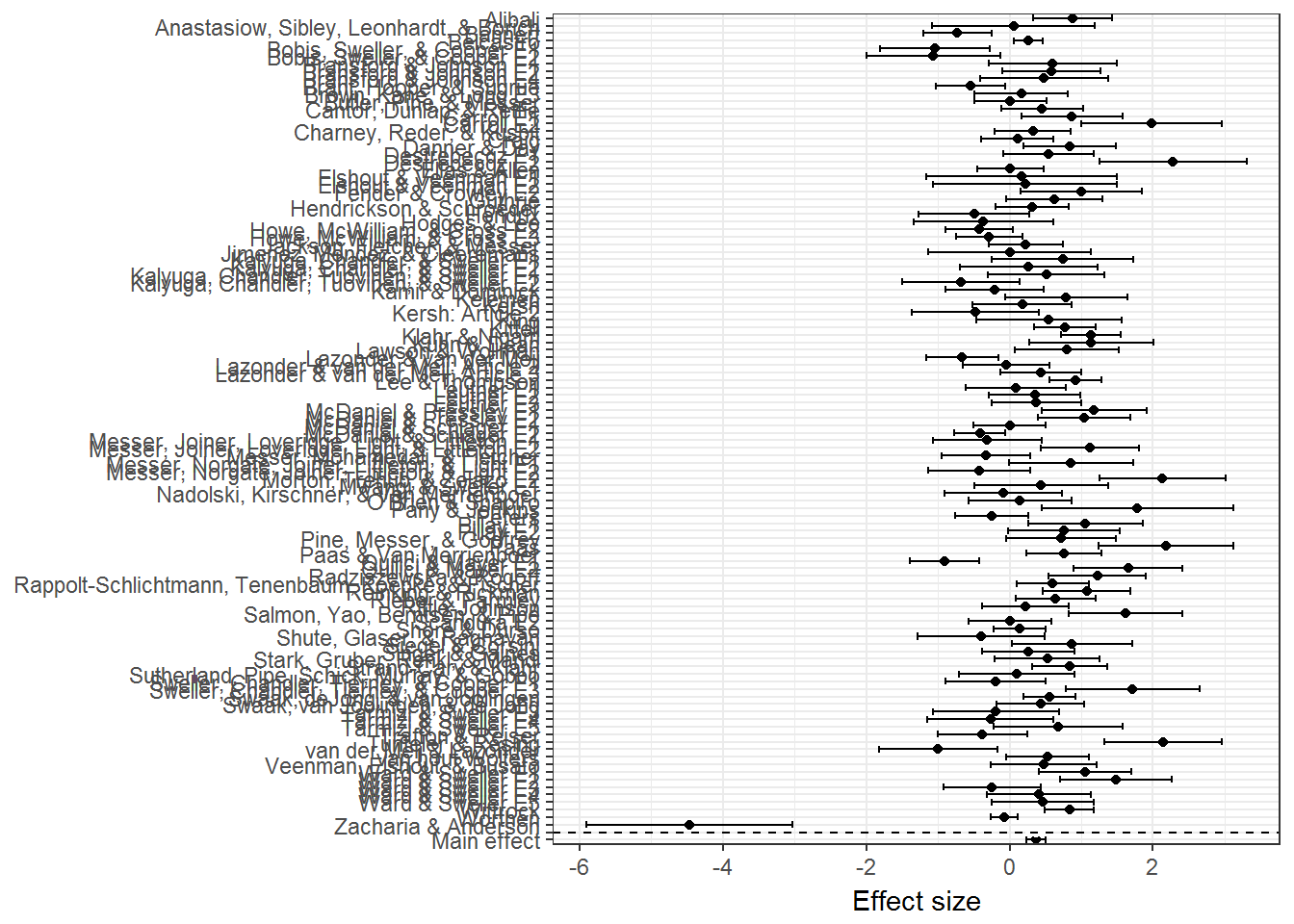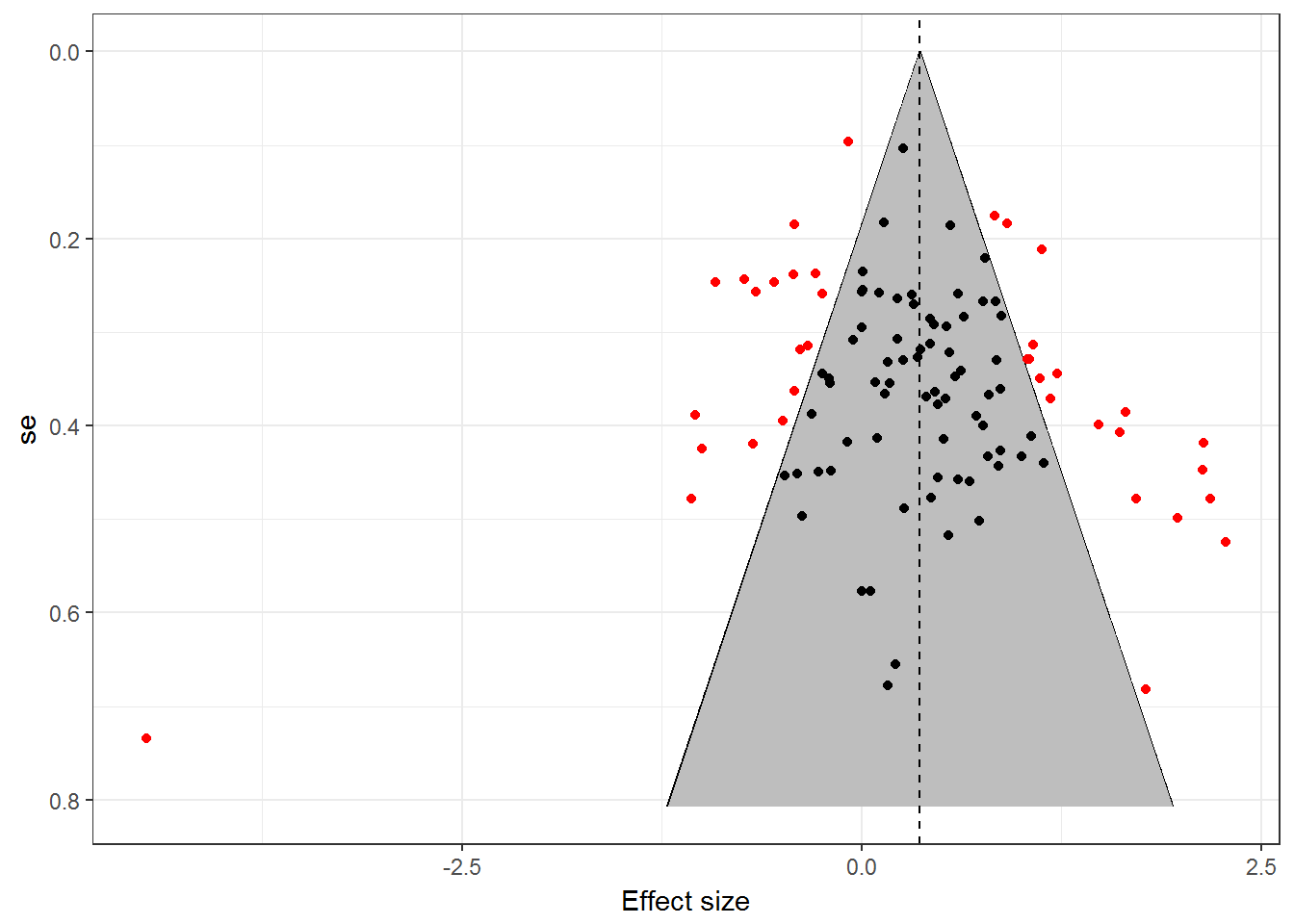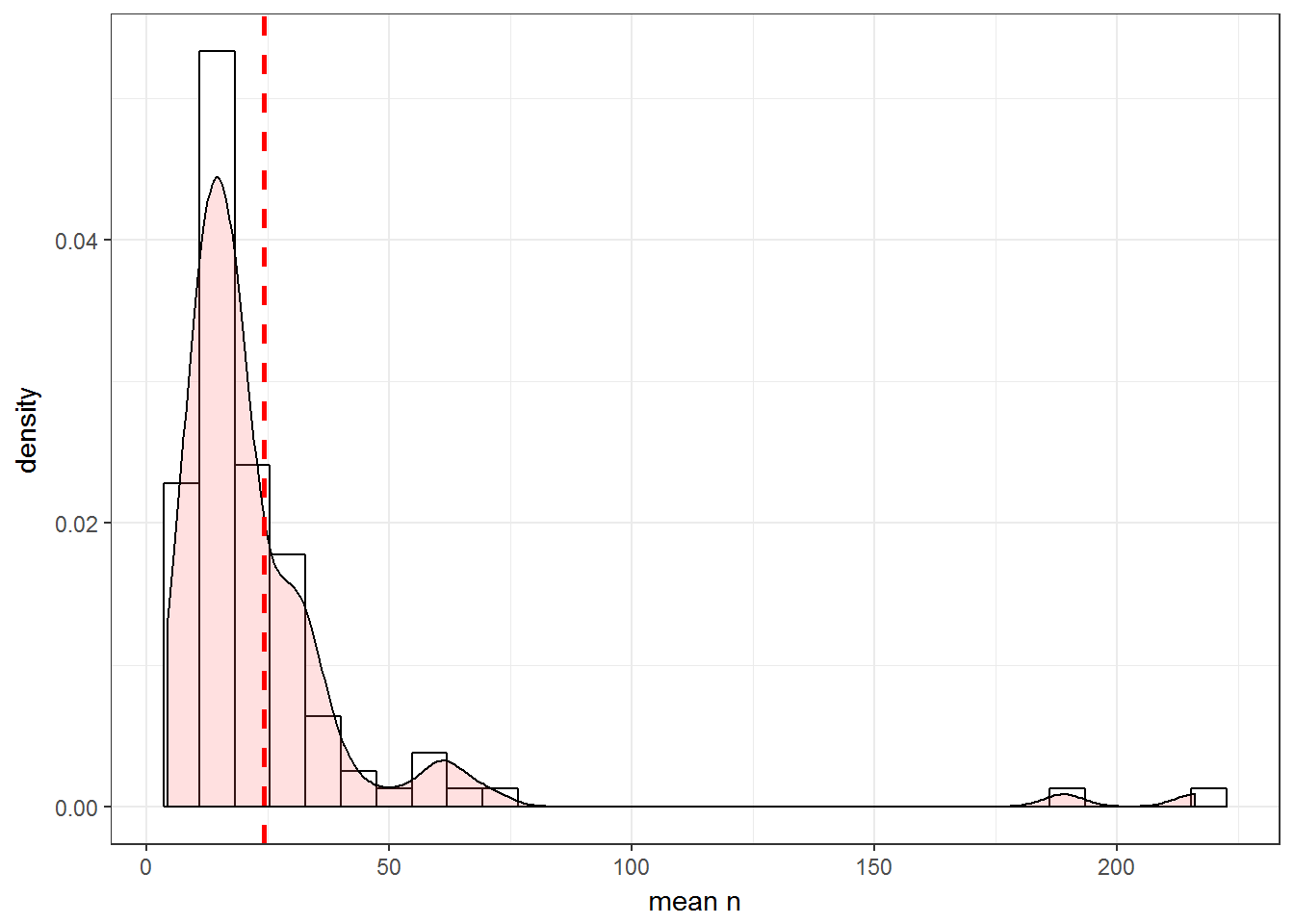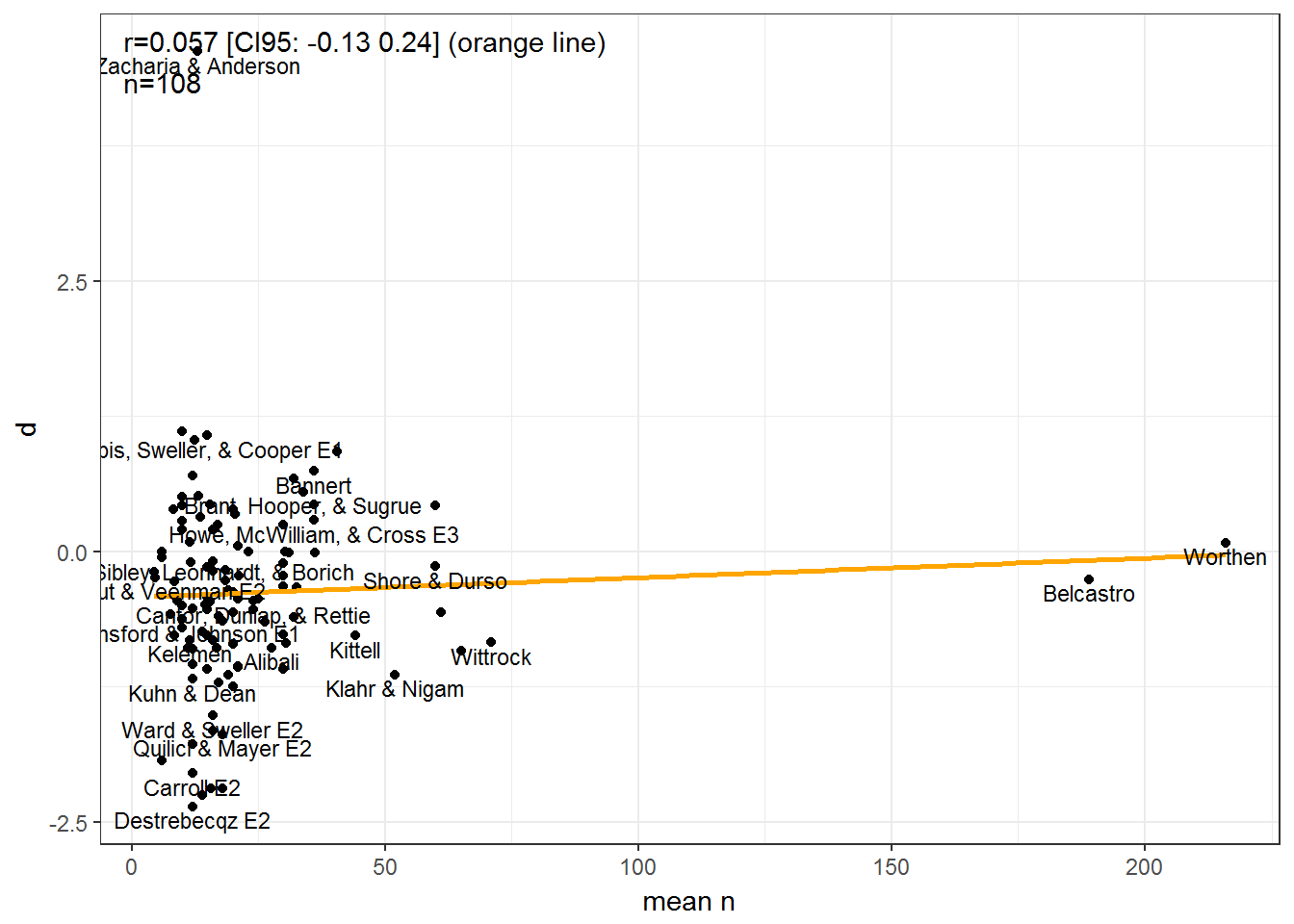# Introduction

We check Alfieri et al (2011) – Does discovery-based instruction enhance learning? – for publication bias. The data are given in the paper’s appendix (PDF). I used Abbbyy FineReader to extract it with minimal trouble.

# Start

library(pacman)

mutate(zeros = rep(0, n()),
ones = rep(1, n()),
mean_n = (Discovery_n + Comparison_n) / 2)
## Parsed with column specification:
## cols(
##   Study = col_character(),
##   Year = col_integer(),
##   Discovery_n = col_double(),
##   Comparison_n = col_double(),
##   d = col_double(),
##   Domain = col_character(),
##   Age = col_character(),
##   Journal_rank = col_character()
## )

# Random effects meta-analysis

The authors did not report the standard deviations or standard errors, only sample sizes and effect size in d. However, given the standardized effect size, we can assume each sample has an SD of 1, and that one of them has a mean of zero, and the other has the d value.

#meta-analyze
meta_main = d_meta %$% rma(m1i = zeros, m2i = d, sd1i = ones, sd2i = ones, n1i = Discovery_n, n2i = Comparison_n, measure = "SMD") %T>% print ## ## Random-Effects Model (k = 108; tau^2 estimator: REML) ## ## tau^2 (estimated amount of total heterogeneity): 0.4100 (SE = 0.0734) ## tau (square root of estimated tau^2 value): 0.6403 ## I^2 (total heterogeneity / total variability): 82.05% ## H^2 (total variability / sampling variability): 5.57 ## ## Test for Heterogeneity: ## Q(df = 107) = 485.8824, p-val < .0001 ## ## Model Results: ## ## estimate se zval pval ci.lb ci.ub ## 0.3665 0.0709 5.1722 <.0001 0.2276 0.5054 *** ## ## --- ## Signif. codes: 0 '***' 0.001 '**' 0.01 '*' 0.05 '.' 0.1 ' ' 1 The original study reported a mean effect of .38 and we get 0.37, close enough that I won’t complain. Discrepnacy may be due to my assumption about the equal SDs in the two groups. # Visualize the data Plot the data using forest and funnel plots. #forest GG_forest(meta_main, .names = d_meta$Study)#funnel
GG_funnel(meta_main)#sample sizes
GG_denhist(d_meta, "mean_n")
## stat_bin() using bins = 30. Pick better value with binwidth.There seems to be some missing left-side studies.

The outlying study is not a data error, at least, it is given as that in the data appendix. It had a sample size of 2x 13, so pretty much any result can happen.

The distribution of sample sizes is skewed and the mean (per group) is very small, 24.19. The median is 16.9.

# Three tests of publication bias

I have 3 easy tests of publication bias available: the two applicable variants of trim and fill, as well as just the correlation.

#trim and fill
trimfill(meta_main, estimator = "L0")
##
## Estimated number of missing studies on the left side: 18 (SE = 6.8142)
##
## Random-Effects Model (k = 126; tau^2 estimator: REML)
##
## tau^2 (estimated amount of total heterogeneity): 0.6635 (SE = 0.1013)
## tau (square root of estimated tau^2 value):      0.8145
## I^2 (total heterogeneity / total variability):   87.51%
## H^2 (total variability / sampling variability):  8.00
##
## Test for Heterogeneity:
## Q(df = 125) = 722.5073, p-val < .0001
##
## Model Results:
##
## estimate       se     zval     pval    ci.lb    ci.ub
##   0.1531   0.0799   1.9163   0.0553  -0.0035   0.3098        .
##
## ---
## Signif. codes:  0 '***' 0.001 '**' 0.01 '*' 0.05 '.' 0.1 ' ' 1
trimfill(meta_main, estimator = "R0")
##
## Estimated number of missing studies on the left side: 0 (SE = 1.4142)
## Test of H0: no missing studies on the left side: p-val = 0.5000
##
## Random-Effects Model (k = 108; tau^2 estimator: REML)
##
## tau^2 (estimated amount of total heterogeneity): 0.4100 (SE = 0.0734)
## tau (square root of estimated tau^2 value):      0.6403
## I^2 (total heterogeneity / total variability):   82.05%
## H^2 (total variability / sampling variability):  5.57
##
## Test for Heterogeneity:
## Q(df = 107) = 485.8824, p-val < .0001
##
## Model Results:
##
## estimate       se     zval     pval    ci.lb    ci.ub
##   0.3665   0.0709   5.1722   <.0001   0.2276   0.5054      ***
##
## ---
## Signif. codes:  0 '***' 0.001 '**' 0.01 '*' 0.05 '.' 0.1 ' ' 1
#correlation
GG_scatter(d_meta, "mean_n", "d", case_names_vector = "Study")So, one variant of trim and fill finds substantial bias (18 missing studies), the other variant finds no bias and neither does the correlation if we judge by the confidence interval’s relationship to 0. However, it can be seen that the largest study found no effect, and the one slightly smaller than it, found a small effect.

The pessimistic conclusion from this is that we don’t really know what works because the published data is very heterogenous (I^2 = 82), there’s signs of publication bias and the studies were almost all very small.